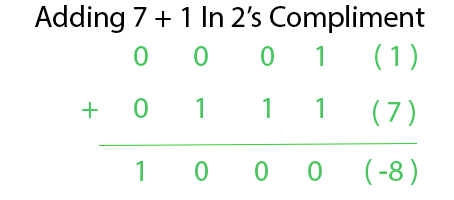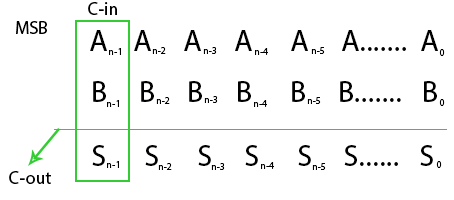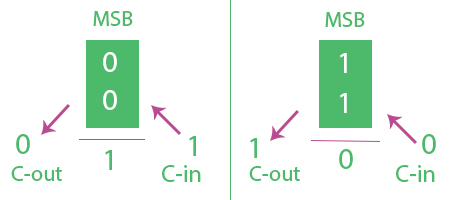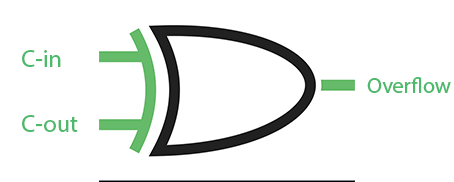Binary Number System | Overflow in Arithmetic Addition

In computer Architecture 2’s Compliment Number System is widely used. The discussion of overflow here mainly will we with respect to 2’s Complimentary System.

N-bit 2’s Compliment number System can represent Number fromto4 Bit can represent numbers from ( -8 to 7 )
5 Bit can represent numbers from ( -16 to 15 ) in 2’s Complimentary System.

Overflow Occurs with respect to addition when 2 N-bit 2’s Compliment Numbers are added and the answer is too large to fit into that N-bit Group.
A computer has N-Bit Fixed registers. Addition of two N-Bit Number will result in max N+1 Bit number. That Extra Bit is stored in carry Flag. But Carry does not always indicate overflow.Adding 7 + 1 in 4-Bit must be equal to 8. But 8 cannot be represented with 4 bit 2’s complement number as it is out of range. Two Positive numbers were added and the answer we got is negative (-8). Here Carry is also 0. It is normally left to the programmer to detect overflow and deal with this situation.

Overflow Detection –
Overflow occurs when:

1. Two negative numbers are added and an answer comes positive or
2. Two positive numbers are added and an answer comes as negative.

So overflow can be detected by checking Most Significant Bit(MSB) of two operands and answer. But Instead of using 3-bit Comparator Overflow can also be detected using 2 Bit Comparator just by checking Carry-in(C-in) and Carry-Out(C-out) from MSB’s. Consider N-Bit Addition of 2’s Compliment number.Overflow Occurs when C-inC-out. Above expression for overflow can be explained from below Analysis.In first Figure the MSB of two numbers are 0 which means they are positive. Here if C-in is 1 we get answer’s MSB as 1 means answer is negative (Overflow) and C-out as 0. C-inC-out hence overflow.

In second Figure the MSB of two numbers are 1 which means they are negative. Here if C-in is 0 we get answer MSB as 0 means answer is positive(Overflow) and C-out as 1. C-inC-out hence overflow.
Readers can also try out other combination of c-in c-out and MSB’s to check overflow.

So Carry-in and Carry-out at MSB’s are enough to detect Overflow.Above XOR Gate can be used to detect overflow.My Personal Notes arrow_drop_upCheck out this Author's contributed articles.

If you like GeeksforGeeks and would like to contribute, you can also write an article using contribute.geeksforgeeks.org or mail your article to contribute@geeksforgeeks.org. See your article appearing on the GeeksforGeeks main page and help other Geeks.

Please Improve this article if you find anything incorrect by clicking on the "Improve Article" button below.

Be the First to upvote.

Please write to us at contribute@geeksforgeeks.org to report any issue with the above content.